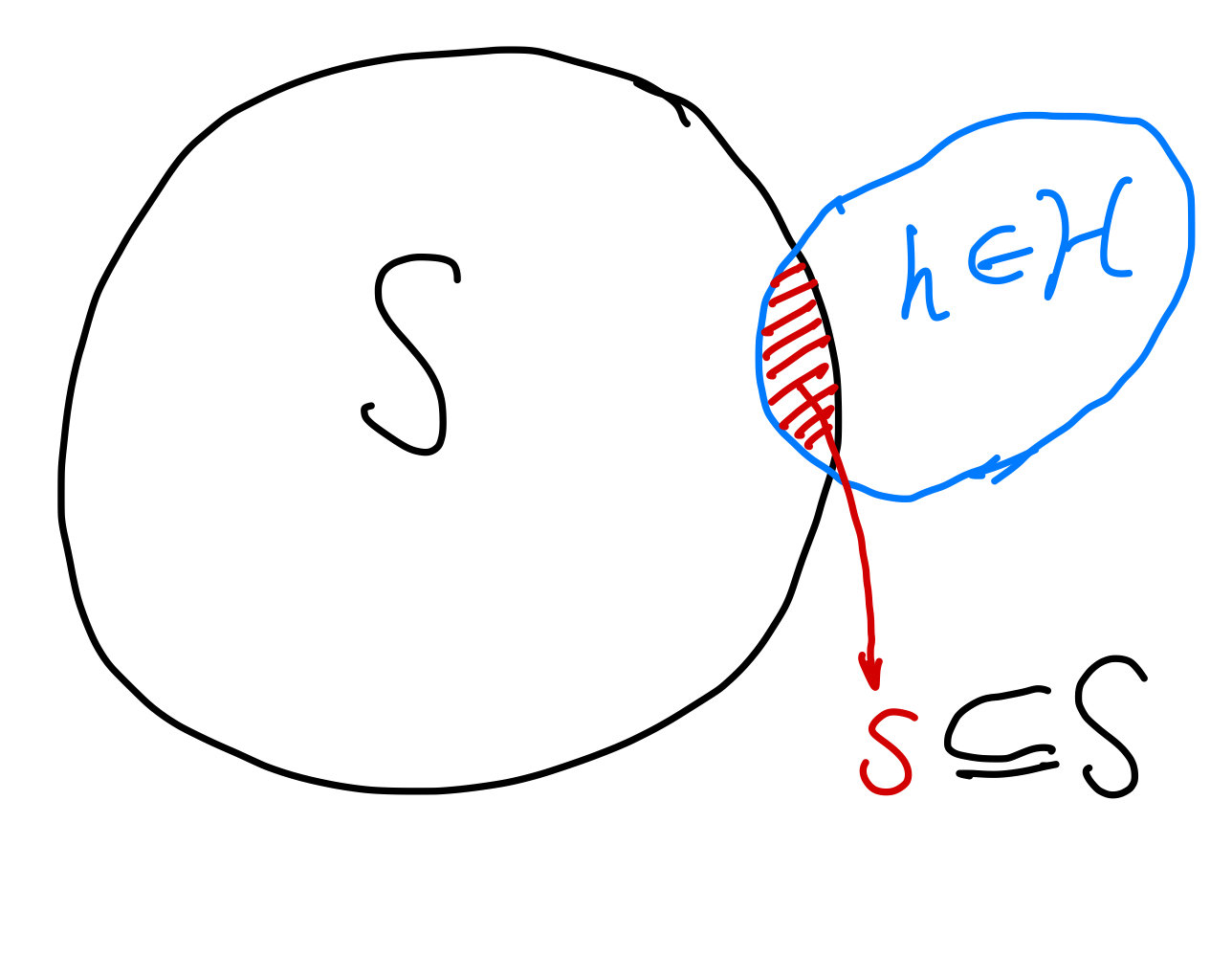Given a set $\mathcal S$, and a class (collection of sets) $\mathcal H$.

For any subset of $\mathcal S$, denoted as $\mathcal s$, if we have an element of class $\mathcal H$, denoted as $\mathcal h$, that leads to1

$$\mathcal h \cap \mathcal S = \mathcal s.$$

Since the power set of $\mathcal S$ ($P(\mathcal S)$) contains all the possible subsets of $\mathcal S$, we can also rephrase the concept using power set. If we can find the power set $P(\mathcal S)$ by looking into intersections of elements $\mathcal h$ of $\mathcal H$ ($\mathcal h\in \mathcal H$), then we say $\mathcal H$ shatters $\mathcal S$ 1.Set $\mathcal S$ is shattered by class $\mathcal H$ if we can generate all possible subsets of $\mathcal S$ (power set of $\mathcal S$).

Planted: by ;

L Ma (2021). 'Shatter', Datumorphism, 10 April. Available at: https://datumorphism.leima.is/cards/machine-learning/learning-theories/set-shatter/.